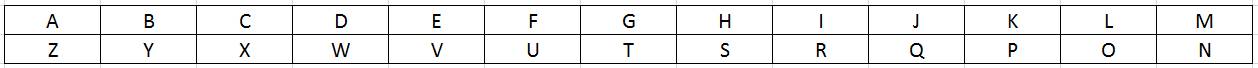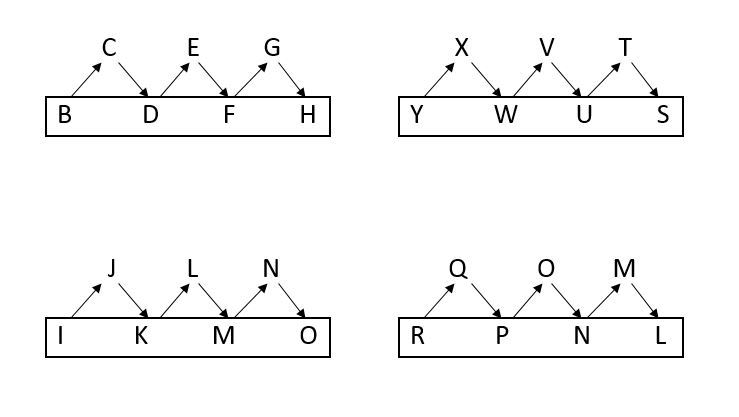0
913

# Analogy Questions for SSC CHSL PDF:

SSC CHSL Analogy Questions download PDF based on previous year question paper of SSC CHSL exam. 25 Very important Analogy questions for SSC CHSL Exam.

SSC CHSL Study Material (FREE Tests)Question 1: ABC: GHI :: OPQ: ?

a) VWX
b) STU
c) UVW
d) RST

Question 2: BEHI : YVSR :: GCMJ : ?

a) TYMP
b) SXMQ
c) SYNP
d) TXNQ

Question 3: IJK : FGH :: PQR: ?

a) LMN
b) MNO
c) JKL
d) QRS

Question 4: BDFH: YWUS:: IKMO: ?

a) RPNL
b) QOMK
c) XVTR
d) PNLJ

Question 5: Tiger : Tigress :: Bull : ?

a) Calf
b) Ox
c) Buffalo
d) Cow

Question 6: ADIL : ZWRO :: BEFK : ?

a) XRSQ
b) YUVP
c) ZTRQ
d) YVUP

Question 7: Calm : Excite :: Fair: ?

a) Knowledge
b) Up
c) Biased
d) Straight

Question 8: Sun : Earth :: Earth : ?

a) Milky way
b) Jupitar
c) Moon
d) Mars

Question 9: STP : TSO ::PTS : ?

a) QST
b) QSR
c) QRS
d) OST

Question 10: son : father :: father : ?

a) Brother.
b) Grand-father.
c) Uncle.
d) Nephew.Question 11: Stream : Flow :: ? : Stagnant

a) River
b) Pool
c) Canal
d) Tributary

Question 12: DEKL : WVPO :: HICD : ?

a) XWRS
b) SRWX
c) SRXW
d) RSWX

Question 13: Door : Carpenter :: Tap : ?

a) Locksmith
b) Laborer
c) Worker
d) Plumber

Question 14: MAN : OCP :: COT : ?

a) EPV
b) DPU
c) EQV
d) EQU

Question 15: Kitten: Cat:: Girl:?

a) Mother
b) Sister
c) Woman
d) Wife

Question 16: Measuring Tape: Length:: Thermometer:?

a) Energy
b) Temperature
c) Heat

Instructions: In each of the following questions, select the related word/letters/number from the given alternatives.

Question 17: Book : Paper : : Bread : ?

a) Flour
b) Biscuit
c) Cake
d) Butter

Question 18: QDXM : SFYN : : UIOZ : ?

a) PAQM
b) LPWA
c) QNLA
d) WKPA

Question 19: BAD : DDH : : CUT : ?

a) DCV
b) DDX
c) ECW
d) EXX

Question 20: Hot : Cold : : ?

a) Summer : Sun
b) Germs : Disease
c) Light : Dark
d) Thin : BigQuestion 21: CFIL : ABCD : : __?___ : WXYZ

a) YBEH
b) DCBA
c) JHPS
d) XURO

Question 22: EVFU : TGSH : : IRTQ : __?__

a) KWLX
b) PKOL
c) OLPK
d) PKLO

Instructions: In each of the following questions, select the related letter/word/number from the given alternatives :

Question 23: Joy : Delight : : Gloomy : ?

a) Silent
b) Dull
c) Vague
d) Plain

Instructions: Select the related letter/word/number from the given alternatives:

Question 24: CFIL : XURQ : : ORUX : ?

a) ROLI
b) RITO
c) LIFC
d) MJFC

Instructions: In each of the following questions, select the related letter/word/number from the given alternatives :

Question 25: Gulp : Drink : : Rush : ?

a) Jump
b) Hop
c) Go
d) RunA+6 = G
B+6= H
C+6 = I
Similarly, the next set of letters for OPQ is (O+6)(P+6)(Q+6) which is UVW.

Let us write the alphabets from A to Z in two rows as shown below.The alphabet B in row 1 corresponds to Y in row 2.
The alphabet E in row 1 corresponds to V in row 2.
The alphabet H in row 1 corresponds to S in row 2.
The alphabet I in row 1 corresponds to R in row 2.
So the word BEHI is written as YVSR.
Applying the same logic “GCMJ” must be written as “TXNQ”.

Here, F,G,H are the alphabets before I,J,K
Hence, the 3 alphabets before P,Q,R are M,N,O
Hence, MNO is the correct answer.

As per the logic shown in the diagramHence, the Option A is the answer

The female counterpart of “Tiger” is “Tigress”.
In a similar way, the female counterpart of “Bull” is “Cow”.
Hence Option D.

Let us write the alphabets from A to Z in two rows as shown below.The alphabet A in row 1 corresponds to Z in row 2.
The alphabet D in row 1 corresponds to W in row 2.
The alphabet I in row 1 corresponds to R in row 2.
The alphabet L in row 1 corresponds to O in row 2.
So the word ADIL is written as ZWRO.
Applying the same logic “BEFK” must be written as “YVUP”.

‘Excite’ is the opposite of ‘calm’. Smilarly, the opposite of ‘fair’ among the options is ‘biased’.

The relation between Sun and Earth is, Earth revolves around the Sun. Similarly, Moon is the one which revolves around the Earth.

In the given series,
Each letter is following a pattern.
First letter:
S =>T
P =>Q
Second letter :
T=>S
T=>S
Third letter:
P=>O
S=>R
Hence, the next term in the pattern is QSR.

Father is the father of son.
Grand-father is the father of father.

Among the given options only a “pool” has stagnant water without flow.
Hence option B.

Let us write the alphabets from A to Z in two rows as shown below.The alphabet D in row 1 corresponds to W in row 2.
The alphabet E in row 1 corresponds to V in row 2.
The alphabet K in row 1 corresponds to P in row 2.
The alphabet L in row 1 corresponds to O in row 2.
So the word DEKL is written as WVPO.
Applying the same logic “HICD” must be written as “SRXW”.

Carpenter repairs doors and wooden furniture.
Plumber repairs taps.
Hence, Option D is the correct answer.

Skipping one letter after M we get O.
Skipping one letter after A we get C.
Skipping one letter after N we get P.
Thus MAN : OCP is arrived.
Applying the same logic we get COT : EQV.
Hence Option C.

The first term grows into the second term
Hence, the Option C is the answer

The first term measures the second term
Hence, the Option B is the answer

A book is formed by papers, similarly bread is made by flour.

=> Ans – (A)

Expression = QDXM : SFYN

The pattern followed is :

Similarly, for UIOZ :

=> Ans – (D)

The pattern followed is :

Similarly, for CUT :

=> Ans – (D)

Hot and cold are antonyms, among the options, words which are opposite of each other are
= Light : Dark
=> Ans – (C)

Expression = CFIL : ABCD : : __?___ : WXYZ

The pattern followed is :

Similarly, for WXYZ :

=> Ans – (A)

Expression = EVFU : TGSH : : IRTQ : __?__

Pairs of opposite letters are used.
E <-> V and F <-> U
Then, for the second term, U (-1 letter) = T
Again, T <-> G and S <-> H
Similarly, I <-> R and T <-> Q
Thus, Q (-1 letter) = P
$\therefore$ P <-> K and O <-> L
=> IRTQ : PKOL
=> Ans – (B)

Joy and delight are synonyms, which is related to happiness, similarly, the word similar in meaning to gloomy is dull.
=> Ans – (B)

Expression = CFIL : XURQ : : ORUX : ?

Pairs of opposite letters are used.

O -> L
R -> I
U -> F
X -> C

Thus, ORUX : LIFC

=> Ans – (C)